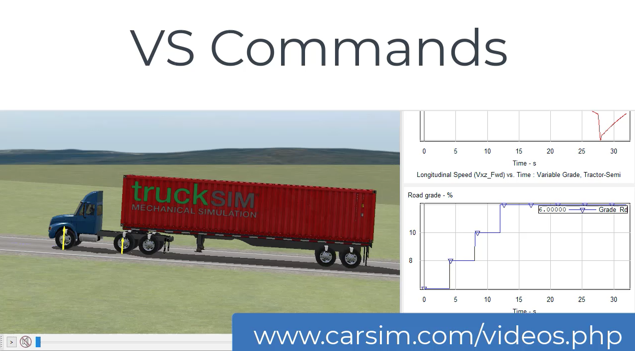This 5-minute video shows how to add features to a model with VS Commands and Event (July 2020).

VS Commands

A VS Math Model reads input files, writes output files, and calculates variables from an internal math model. Besides scanning input files for keywords associated with parameters and tables, a VS Math Model looks for VS Commands that can apply advanced analyses (e.g., Linearization), set up advanced procedures with VS Events, add variables, add equations, and perform other actions.

Here is a two-page summary of VS Commands.

When running a VS Math Model on Windows or a non-RT Linux, it is also possible to enable embedded Python, providing the capabilities of a full programming language.

VS Math Models include a symbolic calculator that allows inputs to be expressed either numerically or in terms of formulas involving other model parameters and variables.

VS Math Models also include a preprocessor with advanced commands that allow users to customize and extend the models by adding equations at run time. Import variables can be assigned to formulas involving other model variables. For example, road friction variables for each tire can be redefined with equations defined at runtime that are based on other variables in the model.

Define New Parameter and Variables

New variables may be defined at run-time for a variety of purposes: import, export and output, constant parameters, and auxiliary variables used in other equations. VS Commands can also be used to add degrees of freedom in the form of new state variables that will be calculated by numerical integration of corresponding user-defined differential equations.

Units

VS Math Models maintain two sets of units. Internal SI units are used in all equations, such the no scale factors are ever used. Angles are radians, lengths are meters, time is seconds, etc. User units are assigned to every variable that is not an integer. By default, these are commonly used units for the associated parameters or variables: angles are degrees, dimensions are mm, vehicle speed is km/h, acceleration is g, etc.

All user units of all parameters and variables can be changed using VS Commands. Further, new units can be defined and assigned to any parameters or variables. For example, forward vehicle speed, normally presented in km/h, is sometimes set for mi/h, m/s, or other units.

New variables added with VS Commands can be calculated with equations that are also defined with VS Commands. The equations can be algebraic, involving all existing and new variables and parameters in the model. Differential equations can also be added; algebraic equations are defined at run time for the derivatives of the new variables.

Equations can be inserted at various times in the simulation: during initialization, at the start of a time step, and the end of a time step, etc.

Equations can also be added for existing import variables that are available for working with external software. Rather than obtaining forces, moments, controls, etc. from external software such as Simulink, they can be defined using equations specified with VS Commands. For example, specify forces and moments to constrain the vehicle sprung mass for simulated K&C tests, such as the video shown at the top of this page.

VS Events

One of the most powerful capabilities is the VS Event. Pending events are set up, in which a variable is monitored and compared to a formula (a number, another variable, or a symbolic expression involving other variables and built-in functions). When the comparison (<, >, =, etc.) is true, then a new data file is loaded that can change almost anything in the model. Go from open-loop to closed-loop control; replace a tire; change roads; reset some vehicle condition; enable and disable writing to file.

Events are commonly used to script complicated test procedures and extend the math model by adding new sensitivities. For example, see the sine with dwell CarSim example.

Linearize

VS Math Models include nonlinear ordinary differential equations (ODEs). These are used to calculate the response of the model to driver controls, interactions with the ground, etc. as functions of time. In normal operation, simulated test results are viewed and analyzed using the same methods as used for physical testing.

There are occasions where classical frequency-domain methods such as Eigenvalues and Bode plotting are helpful. However, these methods are all based on system models with linear equations of motion.

Although the full VS model equations are nonlinear, the behavior of the full system can be approximated as a linear system at any time during a simulation run using perturbation. At any time during the simulation run, the VS Command LINEARIZE can be used to apply a built-in perturbation algorithm to create A, B, C, and D matrices for the linearized system, and write them into a MATLAB M-file. After the simulation run has finished, M-files generated during the run can be loaded into MATLAB for analysis. This feature is commonly used for BikeSim analysis for identifying modes of vibration; as a VS feature it is also available for all time-domain models.

Note: the perturbation method is not valid with HIL systems or models that have been extended in external software that has its own ODEs.

Backing Up In Time to a Saved State

VS Math Models have the built-in capability take a "snapshot" of the current state of the VS Math Model and save it in memory for possible future use. The snapshot consists of the current simulation time, plus the current values of all state variables (including the built-in variables, those added with VS Commands, and the current values of all active Import variables (if there are any). This can be done at constant intervals with the VS Command START_SAVE_TIMER, or only at times of interest, using the VS Command SAVE_STATE when a VS Event is triggered.

Another command RESTORE_STATE is used to restore a saved state. This command is triggered with a VS Event, and is done after changing model parameters to change the behavior for the next try.

The SAVE/RESTORE capability is used internally to perform the perturbations that underlie the LINEARIZE command. The capability is also available for advanced users involved with repetitive situations, such as optimizations or advanced control algorithms. For example, CarSim engineers have worked with race engineers from NASCAR, Formula 1, ALMS, and IndyCar series to set up similar control strategies to limit braking and throttle controls for a turn. If the vehicle can't follow the path, the model jumps back in time and a modified control is attempted.

Embedded Python

When running under Windows OS or non-RT Linux, VS Math Models can use the VS command OPT_ENABLE_PYTHON to enable embedded Python.

With Python loaded, VS parameters and variables (numbers) can be can placed in a table for use as input to the Python routine, and another table can return outputs (also numbers) back for use by other VS Commands. A text string is also available as input to the Python module, and the interpreter itself has full capability for reading and writing files, accessing network resources, etc.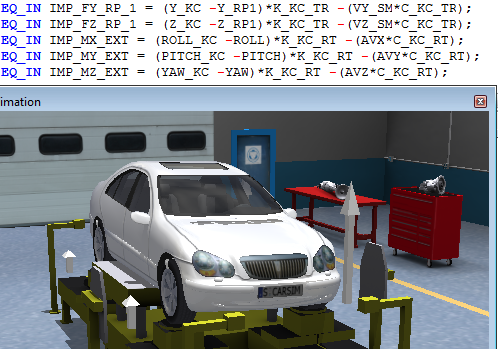VS Commands can add forces and moments to constrain a vehicle for a simulated K&C test. Click for more detail.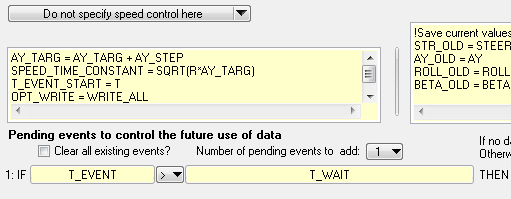VS Events are used for a steady turning test to control the steering input, and to write to output file only after transient responses have settled. The allows cross-plots to be made with only steady-state conditions. Click for more detail.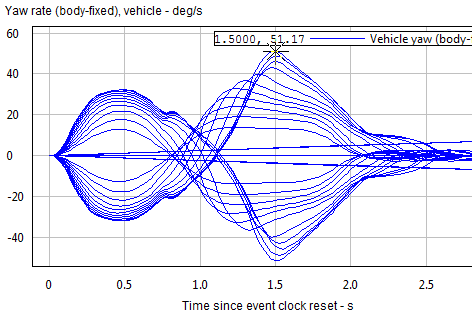VS Events are used make a sequence of sine with dwell tests. These also make use of new custom variables added with VS Commands. Click the figure for more detail.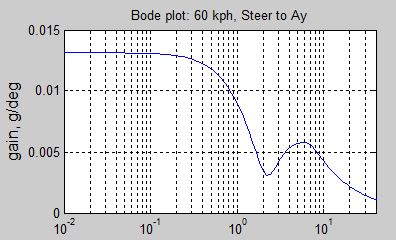The VS Command LINEARIZE generates matrices in an M-File for use in MATLAB. Common applications are Eigenvalues, Eigenvectors, frequency response plots, and root-locus plots. Click the figure for more detail.